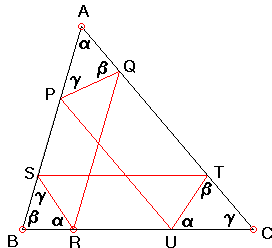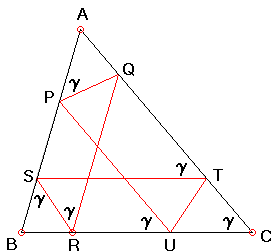# Tucker CirclesWhat Are They? A Mathematical Droodle

ExplanationCopyright © 1996-2018 Alexander Bogomolny

Draw a hexagon inscribed in a given ΔABC with sides successively parallel and antiparallel to the sides of ΔABC. How do you do that? Start with any point on the perimeter and then intermittently draw parallels and antiparallels in a cyclic order. You will always get closed hexagons.Indeed, assume we start with point P and successively draw, antiparallel PQ, parallel QR, antiparallel RS, parallel ST, and antiparallel TU. We want to show that PU is parallel to AC.

The quadrilateral PQRS is a trapezoid with angles P and S equal. Therefore, its sides are also equal: PQ = RS. Similarly, the quadrilateral RSTU is a trapezoid with angles R and U equal. Therefore, RS = TU. By transitivity of equality, PQ = TU.

Now, since PQ and TU are equal and equally inclined to AC, it follows that PU is parallel to AC.

We have to consider another possibility, namely when we began drawing the hexagon with a parallel rather than an antiparallel line. So that assume that we started with U and drew PU, PQ, QR, RS, ST, and TU', where TU' is antiparallel to AB. We'd like to show that U' = U.

Note that the hexagon PQRSTU' starts with the antiparallel PQ. We have just proved that its closing side PU' is parallel to AC. But, by construction PU||AC, from which we get the required equality U' = U.

A curious fact about those hexagons is that they are always cyclic. (A hexagon is cyclic if its vertices lie on a circle.) The circles thus obtained are known as Tucker circles.The isosceles trapezoid PQRS is obviously cyclic. And so is the quadrilateral PSRU, because its opposite angles S and U add up to 180°. Since the two share three vertices (P, R, and U), all five points P, Q, R, S, and U lie on a circle. Three of the four vertices (R, S, U) of the isosceles trapezoid RSTU, which is of course cyclic, lie on that circle. And so does the fourth (T) one. It thus follows that all six points lie on a circle.

### References

1. R. Honsberger, Episodes in Nineteenth and Twentieth Century Euclidean Geometry, MAA, 1995.### Related materialRead more...

• What Is Antiparallel?
• Antiparallel via Three Reflections
• Symmedian and Antiparallel
• Symmedian and 2 Antiparallels
• Circle through the Incenter And Antiparallels
• Batman's Problem
• Antiparallel and Circumradius
•Copyright © 1996-2018 Alexander Bogomolny

 64849286